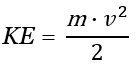# Kinetic Energy Calculator

Easily calculate the kinetic energy of an object in motion. Input supports metric and imperial measurement units. Output in Joules (J, kJ, MJ), Watt-hours (Wh, kWh), calories (Cal, kCal) and foot-pounds (ft-lbs). The calculator can also be used to solve for mass or velocity.

Share calculator:

Embed this tool:
get code

## Using the kinetic energy calculator

This kinetic energy calculator is useful for estimating the energy a body acquires through movement of any kind. For example, it can be used to calculate the kinetic energy of a person, vehicle (car, truck, train), plane, football, as well as for falling bodies that crash into the ground. It can also be used to calculate the energy of different kinds of projectiles. To calculate the kinetic energy you need to know only the body's mass and velocity. Its acceleration does not matter since the energy is calculated at a particular moment of time.

The KE calculator can also be used to solve for any of the other values: mass and velocity. The respective input fields will be hidden or displayed upon selecting what you want to use the calculator for. When solving for mass you need to know velocity and kinetic energy, while when solving for velocity you need to know the object's mass and kinetic energy.

The output of the tool if calculating for kinetic energy is in Joules (J, kJ, MJ), Watt-hours (Wh, kWh), calories (Cal, kCal) and foot-pounds (ft-lbs). When the output of the tool is mass or velocity it is in both standard metric units and imperial ones, with the units automatically being adjusted depending on how big or small the resulting value is.

## Kinetic energy formulas

The formula for kinetic energy (KE) expressed in terms of the body's velocity (speed) (v) and its mass (m) is the first equation below:whereas the second and third ones are the transformed equations for solving for mass or velocity.

This formula is valid in classical mechanics and for non-rotating rigid point objects (the object's mass is assumed to exist at a single point). If the object's speed is larger than a couple of percentages of the speed of light in vacuum it is a poor approximation according to relativistic mechanics. This equation is used in coding this online kinetic energy calculator.

## Kinetic energy calculation examples

Example 1: A car weighs 2200kg (2.2 tonnes) and is moving with a velocity of 40 km/h. What is the car's kinetic energy? To calculate the kinetic energy, first convert km/h to meters per second: 40km/h = 11.11 m/s. Using the first kinetic energy equation above, replace the values for m and v and get KE = 2200 · (11.11)2 / 2 = 135775.3 Joules or 135.7753 kiloJoules.

Example 2: A ball which weighs 500 grams has a kinetic energy of 500 J. What is the velocity of the ball? First we convert 500 grams to 0.5 kg and then replace in the formula: v = √(2 · 500 / 0.5) = 44.7214 m/s or 146.7236 feet per second.

Example 3: A plane is moving at a speed of 800 km/h and it has kinetic energy of 2100 MJ (MegaJoules). What is the plane's mass? First we need to convert MJ to J: 2,100 · 1,000,000 = 2,100,000,000 J. Then we need to convert 800 km/h to m/s which is 222.22(2) m/s. Finally we replace in the formula to get m = 2 · 2,100,000,000 / 222.222 = 85,050 kg = 85.05 tonnes.

Of course, while using our calculator you would not need to perform these unit conversions, as they are handled for you on the fly.

### Ton vs tonne, tons vs tonnes

When solving for the object's mass we output both tonne (metric ton) and ton (short ton). The first one is used by all countries in the world and is defined to be equal to 1000 kg by the international body of standardization. The ton is currently only used in the United States and is equal to 2000 pounds (2000 lbs).